Equations
Exponents
Order of Operations
Fractions & Decimals
Percent
Misc.
100

5x=-105

-21

100

24

16

100

Simplify the following expression: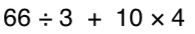100

-4 + -5

9100

Convert the following decimal to a fraction .38

17/50

100

What is 3/4 as a percent?

75%

100

What is the Prime factorization of 20

22*5

200

2p-54=36

45

200

What is 53

125

200

Simplify the following expression: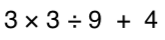200

14 + -12

2

https://www.khanacademy.org/math/arithmetic/arith-review-negative-numbers/arith-review-add-negatives-intro/v/adding-negative-numbers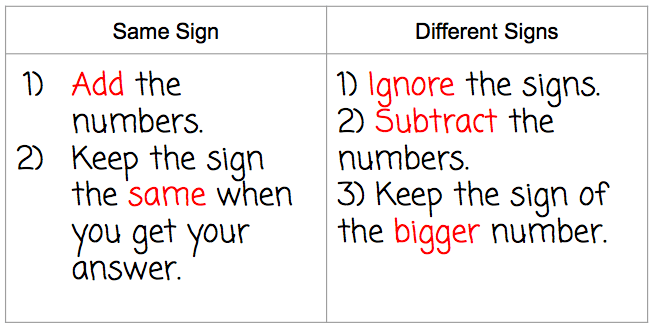200

Multiply 2/3 x 5/4

5/6

200

50% of 297 is what number?

150

200

What is the Prime Factorization of 68

17*22

300

3x+1=-8

x=-3

300

What is 25

32

300

3 + 42 * (3 + 2)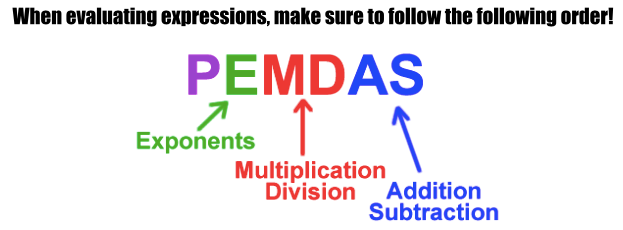300

-10 + 15

5300

Convert the following fraction to a decimal 7/20

.35

300

95% is what as a fraction in simplest form?

19/20

300

What is the greatest common factor of 20, 48

4

400

6+ k/4 = 14

32

400

What is the value of:

83

512

400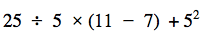400

-18 + (-12) =

-30

400

3/4 + 1/6

11/12

400

What percent of 56 is 224?

400%

400

Find the Least common Multiple of 14, 21 and 7

42

500

27 =5x +2

x=5

500500

6 + (-2 + -5)

-1

500

Divide the following

2/5 divided by 7/10

4/7

500

12.5 is what percent of 1250?

1%

500

Find the Least Common Multiple of 6, 15

30

Click to zoom